@import url(http://www.google.com/cse/api/branding.css);Custom Search The content of the site is exclusively owned by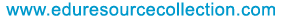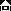Home | School Level | School Projects | Examinations | Civil | Food Processing | Seminars | Software Testing | Warehousing & Inventory | Networking

# Strength of Materials - Stress

2. Stresses
Stress is defined as the internal resistance set up by a body when it is deformed. It is measured in N/m2 and this unit is specifically called Pascal (Pa). A bigger unit of stress is the mega Pascal (MPa).

1 Pa = 1N/m2,
1MPa = 106 N/m2 =1N/mm2.

2.1. Three Basic Types of Stresses
Basically three different types of stresses can be identified. These are related to the nature of the deforming force applied on the body. That is, whether they are tensile, compressive or shearing.

2.1.1. Tensile Stress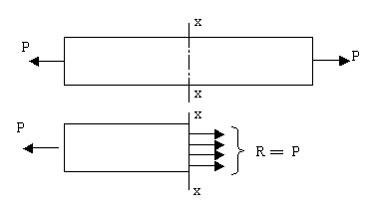Consider a uniform bar of cross sectional area A subjected to an axial tensile force P. The stress at any section x-x normal to the line of action of the tensile force P is specifically called tensile stress pt . Since internal resistance R at x-x is equal to the applied force P, we have,
pt = (internal resistance at x-x)/(resisting area at x-x)
=R/A
=P/A.
Under tensile stress the bar suffers stretching or elongation.

2.1.2. Compressive Stress
If the bar is subjected to axial compression instead of axial tension, the stress developed at x-x is specifically called compressive stress pc.
pc =R/A
= P/A.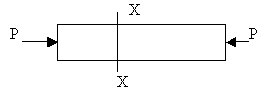Under compressive stress the bar suffers shortening.

2.1.3. Shear Stress
Consider the section x-x of the rivet forming joint between two plates subjected to a tensile force P as shown in figure.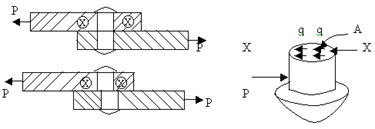The stresses set up at the section x-x acts along the surface of the section, that is, along a direction tangential to the section. It is specifically called shear or tangential stress at the section and is denoted by q.
q =R/A
=P/A.

2.2. Normal or Direct Stresses
When the stress acts at a section or normal to the plane of the section, it is called a normal stress or a direct stress. It is a term used to mean both the tensile stress and the compressive stress.

2.3. Simple and Pure Stresses
The three basic types of stresses are tensile, compressive and shear stresses. The stress developed in a body is said to be simple tension, simple compression and simple shear when the stress induced in the body is (a) single and (b) uniform. If the condition (a) alone is satisfied, the stress is called pure tension or pure compression or pure shear, as the case may be.

2.4. Volumetric Stress
Three mutually perpendicular like direct stresses of same intensity produced in a body constitute a volumetric stress. For example consider a body in the shape of a cube subjected equal normal pushes on all its six faces. It is now subjected to equal compressive stresses p in all the three mutually perpendicular directions. The body is now said to be subjected to a volumetric compressive stress p.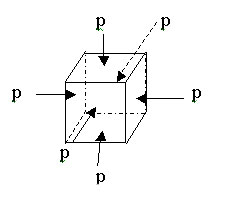Volumetric stress produces a change in volume of the body without producing any distortion to the shape of the body.